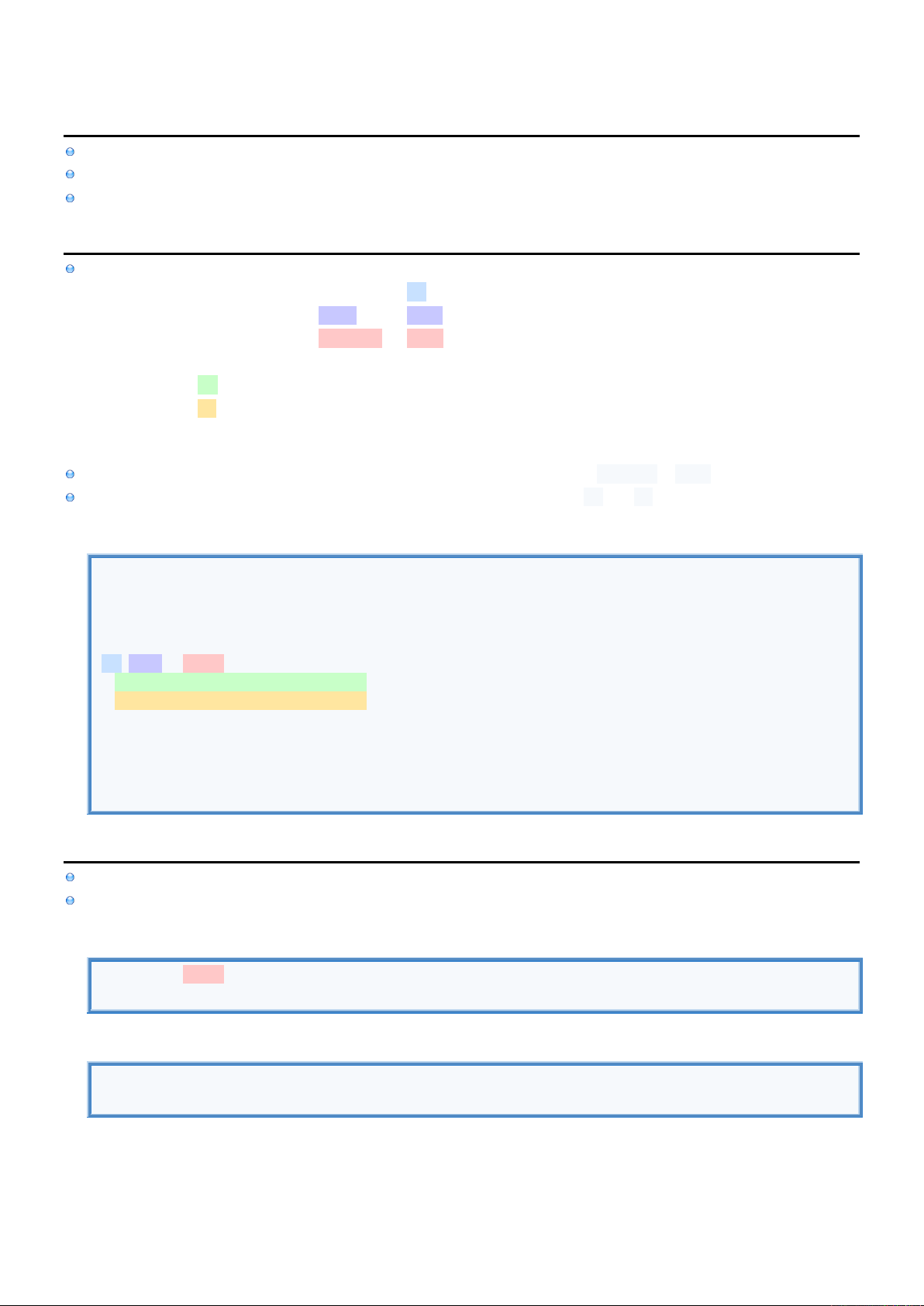2
2
.
.
5
5
.
.
4
4
P
P
r
r
o
o
p
p
e
e
r
r
t
t
i
i
e
e
s
s
-
-
C
C
o
o
m
m
p
p
u
u
t
t
e
e
d
d
I
I
n
n
f
f
o
o
Computed Property uses setter and getter Methods to store and retrieve values from other Variables/Methods.
In other words Computed Property can't store any Values. Instead they are used as a Proxy to other Variables/Methods.
After declaration you use same Syntax for normal Variables which is why you can consider them as Computed Variables.
C
C
o
o
m
m
p
p
u
u
t
t
e
e
d
d
P
P
r
r
o
o
p
p
e
e
r
r
t
t
y
y
S
S
y
y
n
n
t
t
a
a
x
x
Computed Property is declared by
using required Keyword var (Can't be let even if it is read only)
followed by required Variable's Name name
followed by required Variable's Data Type String (Optional only if Value is given from which it can be inferred)
followed by declaration of
required get Method which can be defined without get Keyword if there is no setter
optional set Method if Computed Property is not supposed to be read only
E
E
x
x
a
a
m
m
p
p
l
l
e
e
In this example we declare two normal Variables that will actually hold the data: greetText & name.
Then we declare Computed Property which uses these two Variables inside its get and set Methods.
Computed Property Syntax
//DECLARE VARIABLES.
let greetText : String = "Hello"
var name : String = "" //Normal Variable where value is acctualy stored
//DECLARE COMPUTED PROPERTY.
var greet : String {
get { return "\(greetText) \(name)" } //return can be ommited for single line
set { name = newValue }
}
//REFERENCE COMPUTED PROPERTY.
greet = "John" //Stores "John" into name Variable
print(greet) //Retreives "Hello John" by combining Variables greetText & name
O
O
n
n
l
l
y
y
g
g
e
e
t
t
t
t
e
e
r
r
If Computed Property only has getter it can be omitted.
If getter only has single line return can be omitted.
Implicit getter & return
var greet : String { "Hello John" } //Return can be ommited for single line
print(greet)
Explicit getter & return
var greet : String { get { return "Hello John" } }
print(greet)C
C
o
o
m
m
p
p
u
u
t
t
e
e
d
d
P
P
r
r
o
o
p
p
e
e
r
r
t
t
y
y
r
r
e
e
t
t
u
u
r
r
n
n
s
s
C
C
l
l
o
o
s
s
u
u
r
r
e
e
Highlighted in Red looks like Computes Property has Input Parameters and Return Value.
Actually this is just a Return Value in the form of Closure (that has Input Parameters and Return Value).
Call to myCompProp returns Closure, but since we immediately call this Closure with Parameters it looks like Computed
Property is Closure.
Computed Property returns Closure
//DECLARE VARIABLES.
let greetText : String = "Hello"
var name : String = "" //Normal Variable where value is acctualy stored
//DECLARE COMPUTED PROPERTY.
var myCompProp : (String, Int) -> (String) {
var greetClosure : (String, Int) -> (String) = { name, age in return("\(name) is \(age) years old,") }
return(greetClosure)
}
//REFERENCE COMPUTED PROPERTY.
var result = myCompProp("John", 50) //myCompProp returns Closure. Then we immediately call it.
print(result)